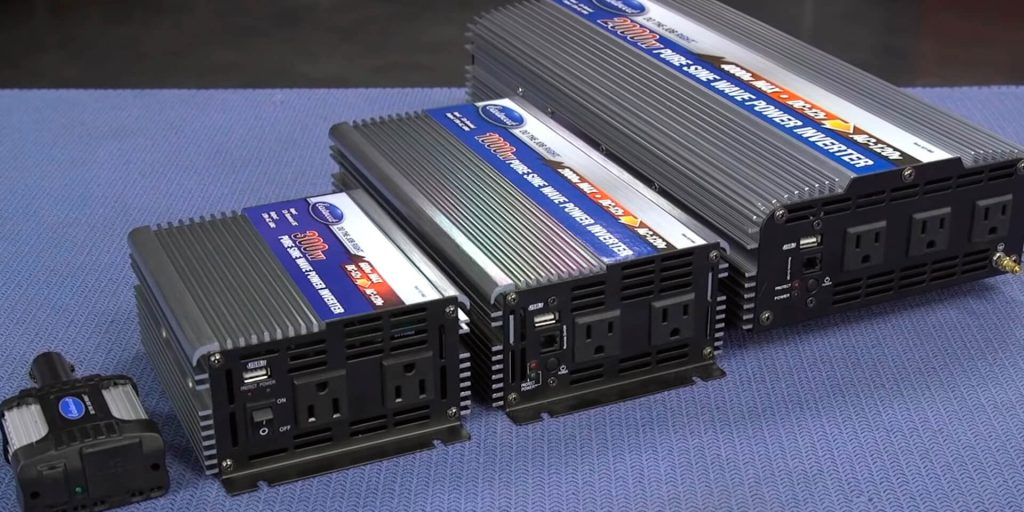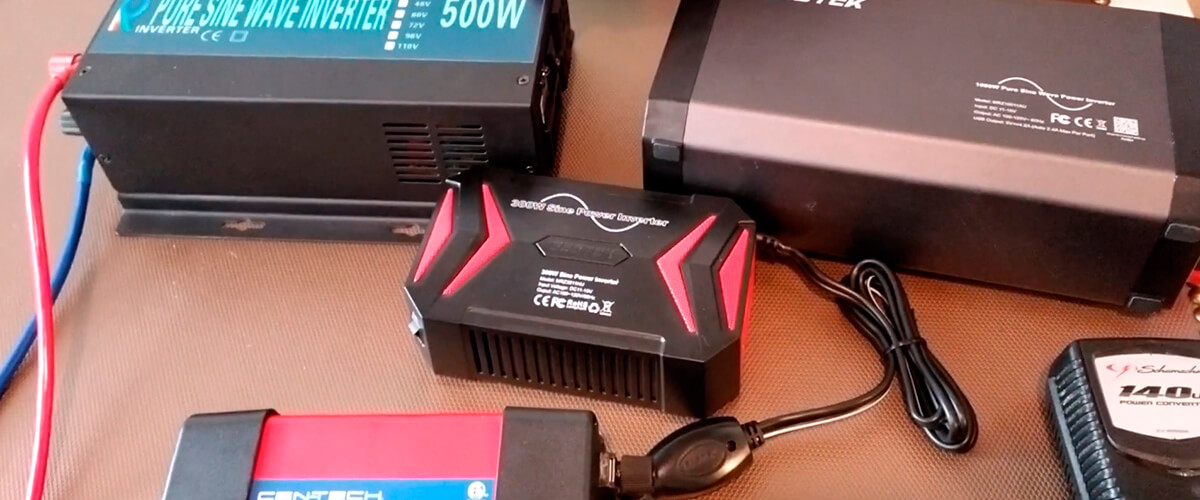# How big an inverter do you needAs you probably already know, an inverter is a device that converts power from a 12-volt DC battery to 120 volts AC.
With it, you can easily power all the electronics in your home. Moreover, if you are interested in what size power inverter do I need, then you have come to the right place. It is very important to choose the size because all further work will depend on it, so I suggest not to hesitate!

## How big of a power inverter do I need?

As we already understood, size plays a very important role. First of all, you should be concerned about the wattage required for all your devices.

Yes, that’s right, you need to roughly imagine what kind of devices you are going to power from your inverter. In addition, there is a general rule for choosing the right inverter. The power that it supplies must be twice as large as the total power of all simultaneously connected devices.

It will be much easier to understand this with an obvious example. It can be assumed that you need to power a PC, a desk lamp, a TV, and, say, a printer in order to work. Now you need to calculate how much each device consumes separately and sum them up into one number. For example, each of these devices consumes exactly 100 watts of power. The total is 400 watts of energy.

Looking at this, we need a size of inverter that will be capable of producing 800 or more watts. In addition, no one cancels the fact that you want to connect with something else in the future. It is because of this that it would be more expedient to buy an inverter, even more, about 1000 W. If you plan on using a lot of electronics, you might even need a 2000 watt inverter.## How to determine the power consumption of the appliance?

To calculate the approximate amount of energy and consumption that you need, you just need to do simple mathematical calculations or calculate the total power by summing up the needs of each device.

For devices with AC or DC adapters, these values ​​are often printed directly on the power supply. In addition, the key formula for calculating this value is a simple equation: Amps x Volts = Watts.

Thus, it is easy to understand that you need to multiply the amperes and volts of a single device and determine its consumption in watts. In addition, you can do even easier and search the power of devices directly on the Internet. In some cases, you can simply watch the power of your device online.

Let’s imagine a hypothetical situation where you want to connect a TV to your car. First, look at your TV’s power source. Suppose the input voltage reads “100-130V”. The current strength is about 5 A. Now, if we use the formula, we get 5 x 110 = 550.

The answer is obvious: you need an inverter with at least 600 watts to turn on the TV in your car without any problems. Thus, the choice of the size of the inverter directly depends on the watts. Moreover, it is worth considering that different devices consume different amounts of energy, depending on what you are doing. A clear example is consoles, which consume significantly less in stand-by mode than in active game mode.

## What are peak and continuous load?

When choosing an inverter, another important thing that determines its size is the difference between constant and peak output power. However, it is important to take into account that the peak power is the power that the inverter supplies for short periods of time during consumption surges, for example, in the first seconds of start-up.

Continuous power is the limit for normal operation. Let’s say your devices consume a total of about 1000 watts, so you need to choose an inverter with a constant output power of 1000 watts.